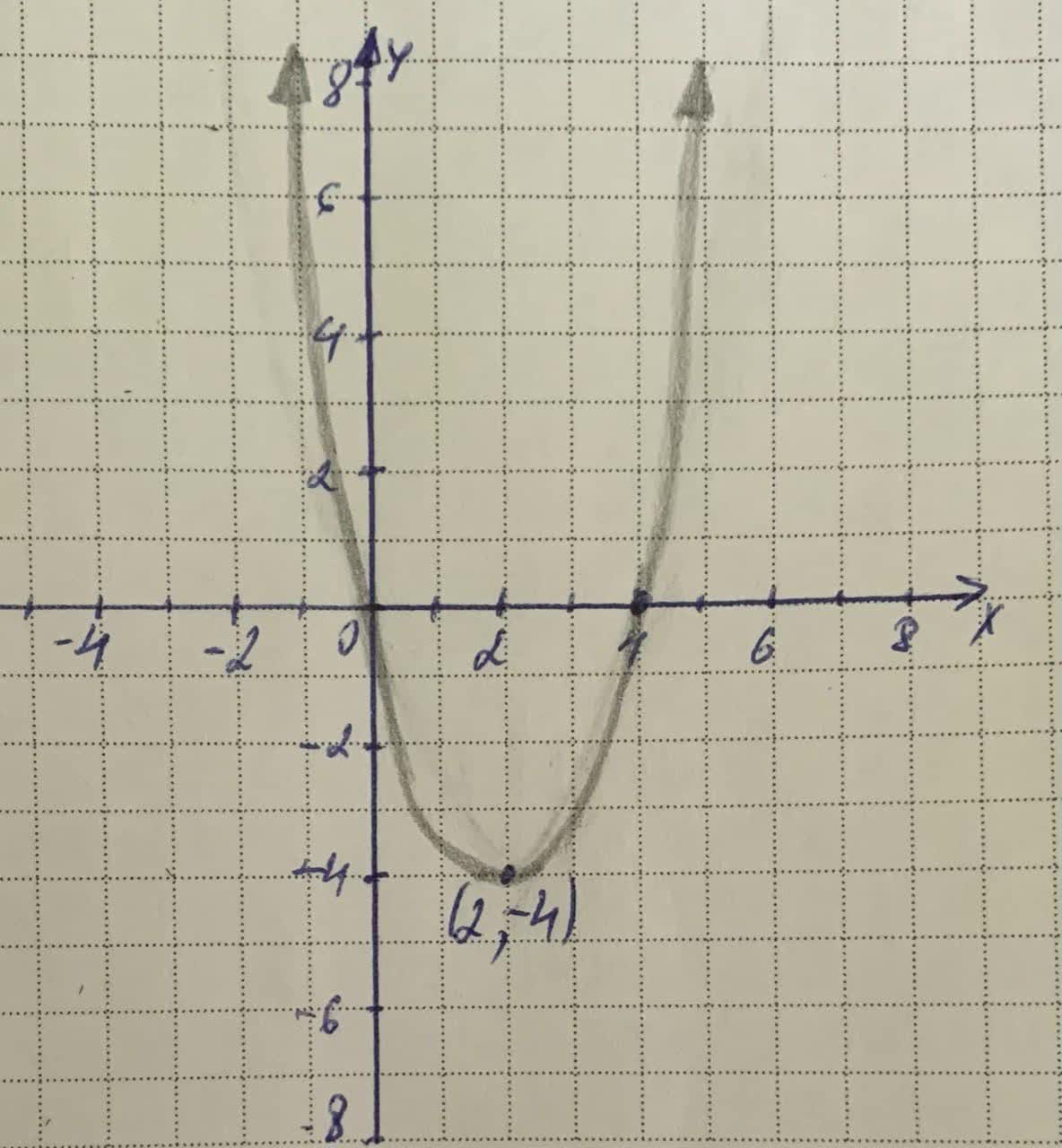pro4ph5e4q2

2021-12-02

Write the equation of the quadratic function whose graph is shownJulie Mathew

Step 1
To find the equation of a quadratic function whose graph is,
shown and write it in the form
$f\left(x\right)=a{x}^{2}+bx+c$
A quadratic function fin vertex form is written as
$f\left(x\right)=a{\left(x-h\right)}^{2}+k$
where h and kare the x and y coordinates plot in the graph
Step 2
$y=a{\left(x-h\right)}^{2}+k,h=2,k=-4,x=4,y=0$
$⇒0=a{\left(4-2\right)}^{2}+\left(-4\right)$
$0=a{\left(2\right)}^{2}-4$
$0=a4-4$
$a4=4$
$a=1$
$f\left(x\right)=1{\left(x-2\right)}^{2}-4$
$=1\left({x}^{2}-2x+4\right)-4$
$={x}^{2}-2x+4-4$
$f\left(x\right)={x}^{2}-2x$

Do you have a similar question?